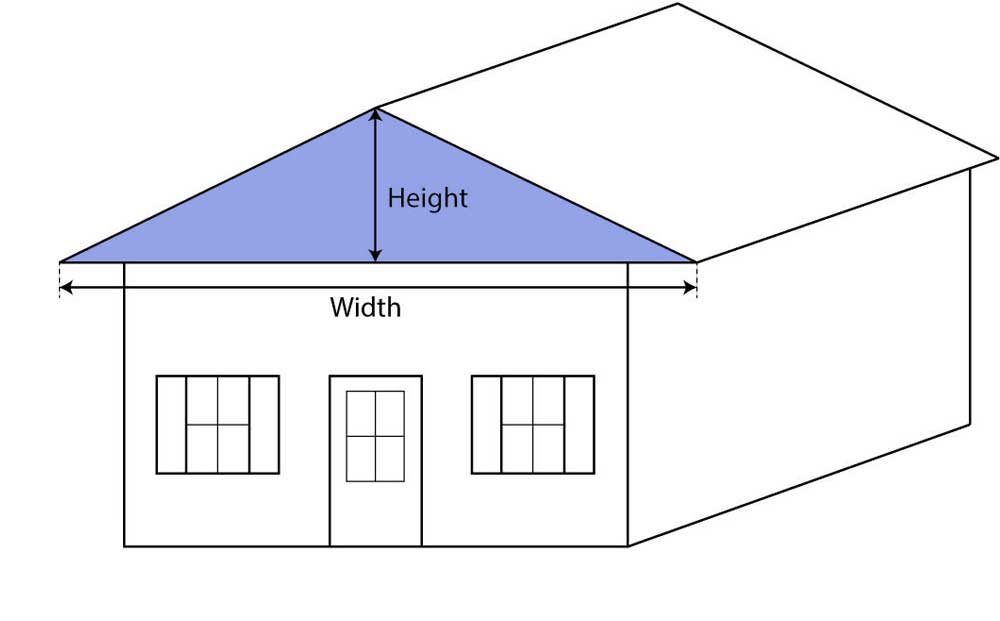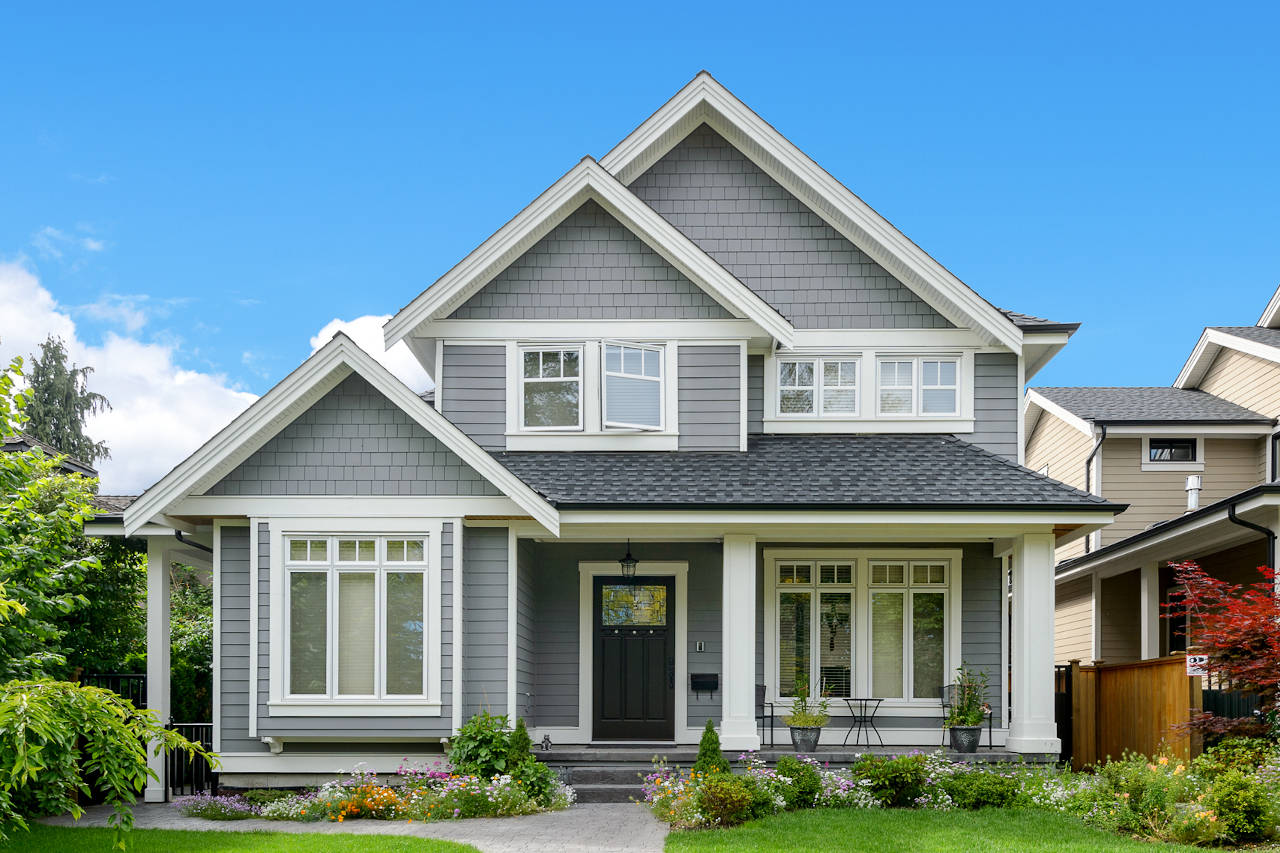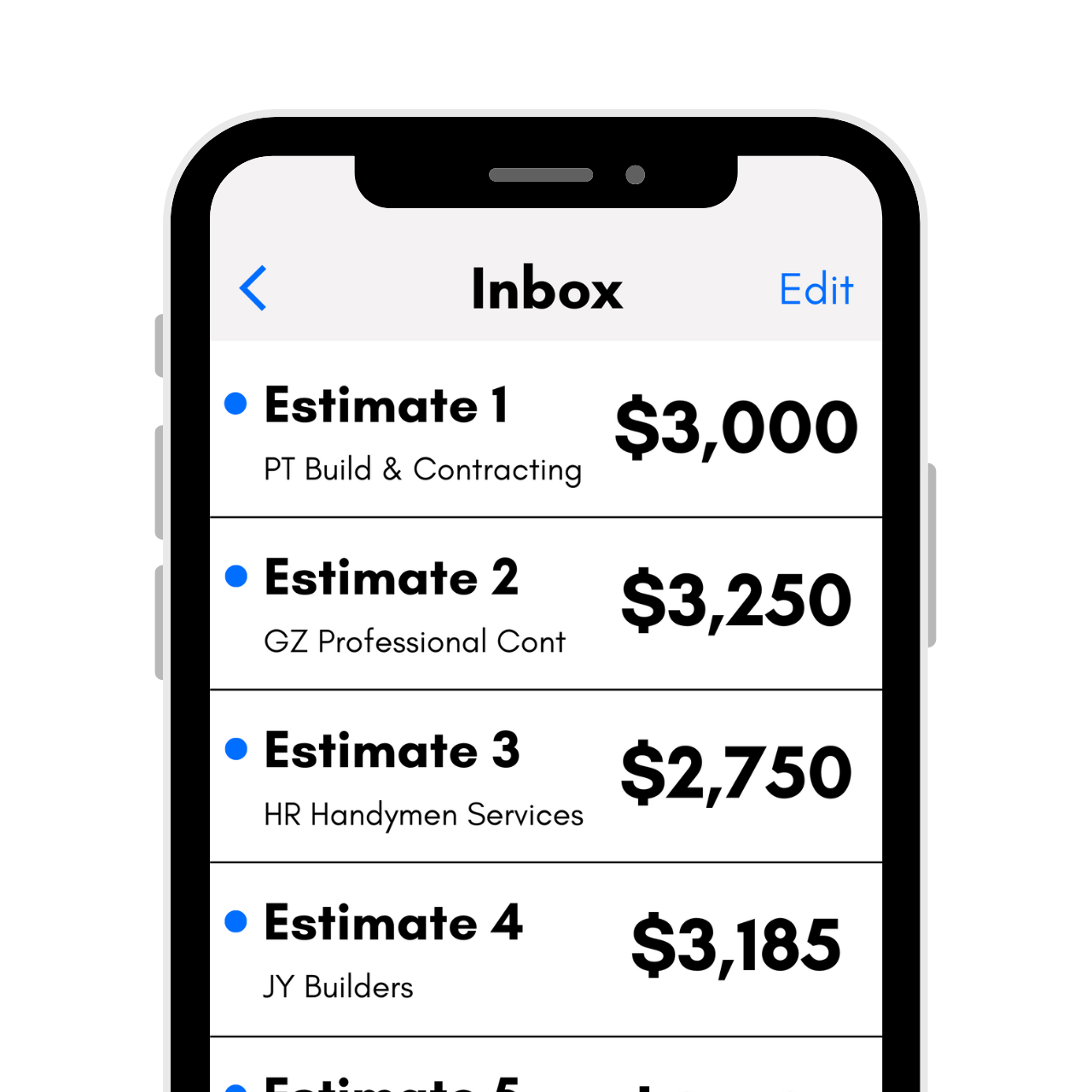# Siding Material Calculator

Find the number of squares of siding material needed by entering the dimensions of the building below for a wall or gable. Optionally, include the number of doors and windows to refine the estimate.## Results:

Material Needed

Squares

ft2
Learn how we calculated this below

## How to Measure for SidingIf you’re planning a siding replacement project or preparing to install a new exterior, take the time to estimate the size of the project as your first step. Doing so will help with estimating material and labor costs.

While different types of siding may come in different styles and may be installed in varying ways, they all require correct measurements for the best results.

### How Much Is a Square of Siding

Siding is often estimated and priced by the square. A Square is a term used by contractors, installers, and building suppliers and is a unit of measure equal to 100 square feet of material.

Most popular types of siding, like vinyl siding, are commonly estimated by the square to determine how much labor and material is needed.

One square of siding will cover 100 square feet. Siding may be packaged and sold in varying quantities of squares. For example, an average box of vinyl siding contains two squares of material.To find the number of squares of siding needed for an installation, measure the width and height of each wall in feet. Multiply the width and height to get the area of the wall in square feet.

If your walls are in a mixture of partial and total feet, round up to the nearest whole foot. For example, if one wall is 12.4 feet and one is 20.8 feet, round to 13 and 21 feet, respectively, before multiplying.

Divide the square footage by 100 to get the number of squares needed for the wall.

For example, a wall that is 40 ft wide and 12 ft high will require at least 4.8 squares of material.

40 × 12 = 480
480 ÷ 100 = 4.8

Be sure to round up to the nearest square, so in this case, you’d probably need 5 full squares.

## How to Measure Gable Walls

Finding the square footage of a gable wall is similar to finding the square footage of a standard wall. Measure the width of the gable and the height of the gable from the base of the triangle to the peak.

Then multiply the width and height, just like you would to find the area of a rectangle. Finally, divide the area in half to get the final square footage. Divide by 100 to get the number of squares.

For example, a 20ft wide gable that is 10ft tall will require 1 square of material.

20 × 10 = 200
200 ÷ 2 = 100
100 ÷ 100 = 1

It is a good idea to order additional material to account for angle cutoffs along the edge of the gable roof.

If you have a very steep gable, such as a salt box or an A-frame, or you have a very tall home, you can get more specific measurements if desired. Measure from the ground to the lowest edge of the roof to get the height, then straight across to get the width.

This will give you the square feet of the wall below the gable. For the gable itself, you want the height from the lowest point of the roof to the highest, as well as the total width. Take the total width and divide it in half, then multiply by the height to get the square footage of the gable.

Add it to the square footage of the area below the gable to get the total square footage of the wall.

## How to Account for Doors and Windows

A wall with doors and windows may require fewer squares of material to cover than a wall with no openings. To account for this overage in material, find the square footage of space that will not be covered in siding for the doors and windows and subtract that from the total square footage of the wall.

For example, an average door is 3 ft wide by 7 ft high. Subtract 21 sq ft from the total square footage to account for a door. Windows vary in size, and the square footage can be found by multiplying the length and the width.

## Material Overages

Because there will inevitably be some waste due to off-cuts, measurement errors, or unintended damage, it’s always a good idea to order some extra material for the project. It is commonly recommended to order an additional 10% material to account for waste.

Having left over siding material is also a good idea since it allows you to make future repairs that will match the existing siding.